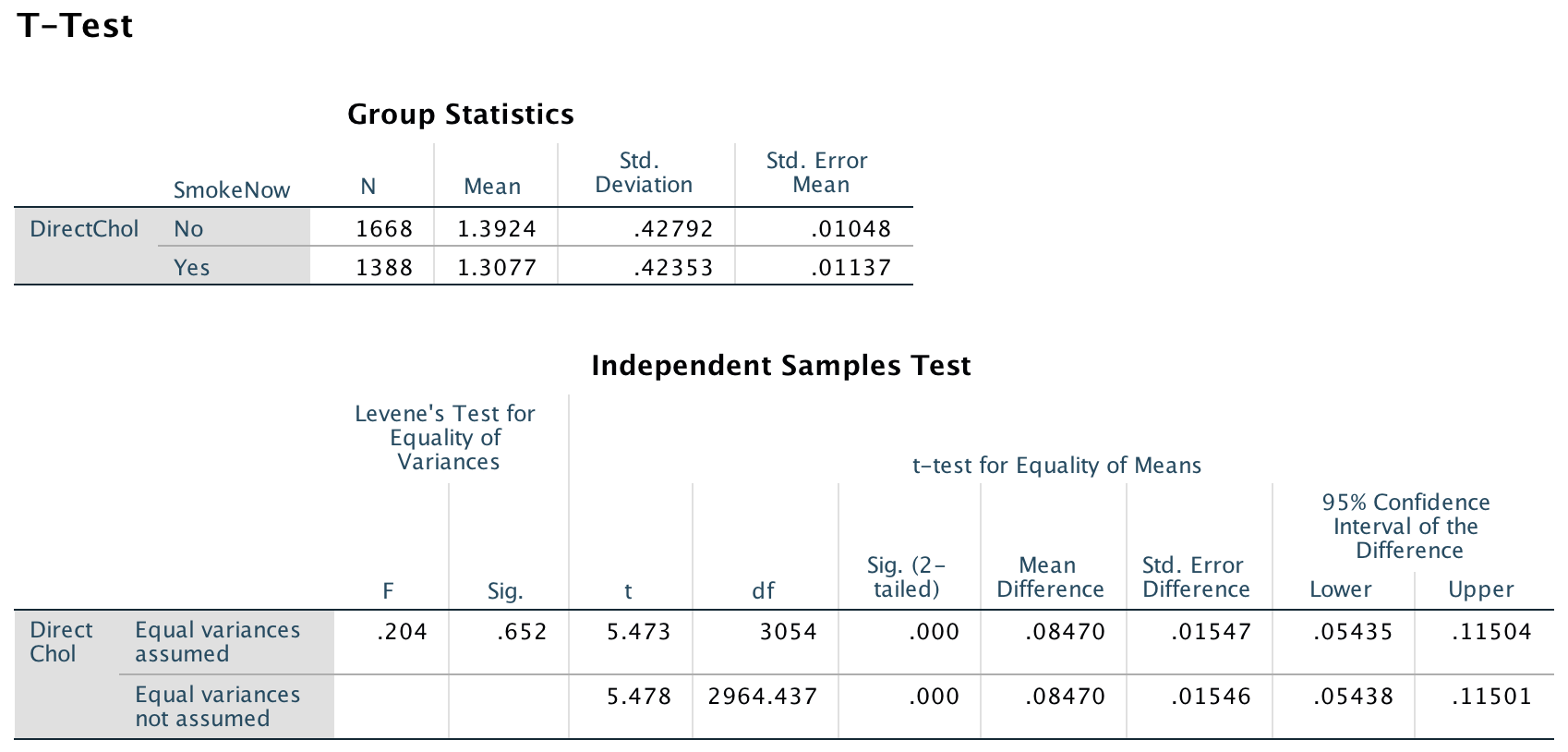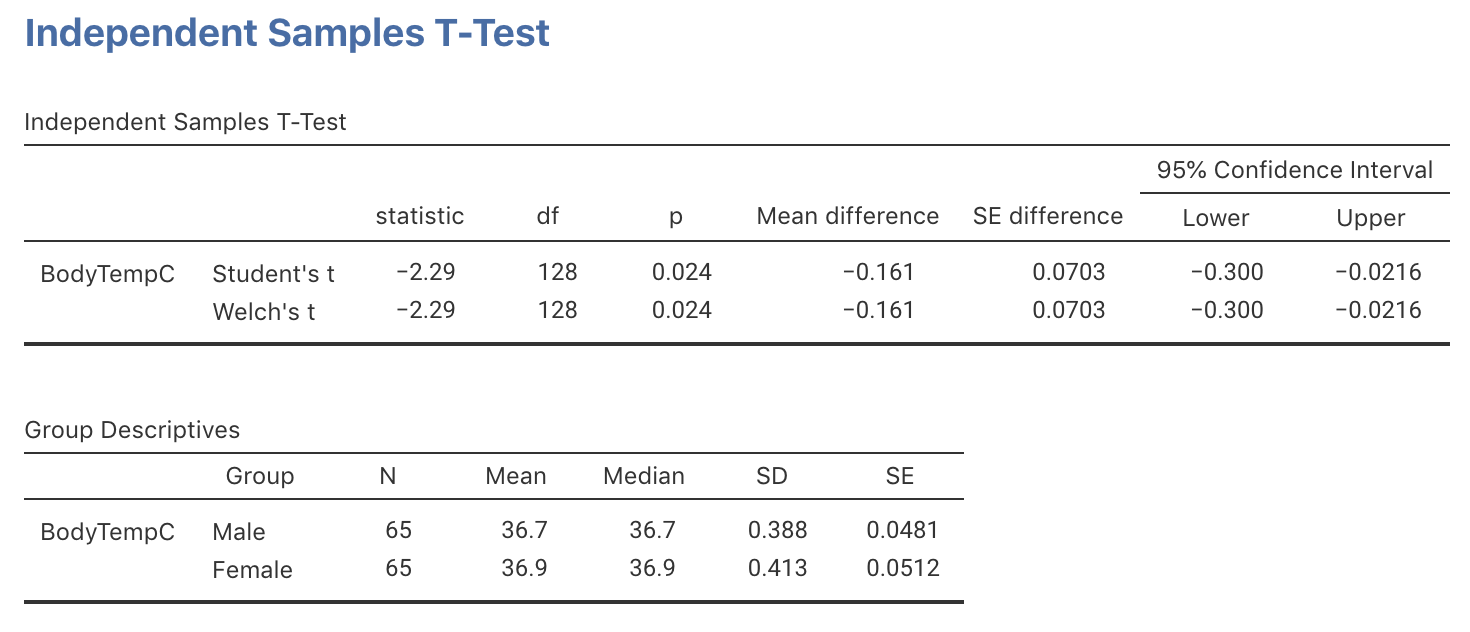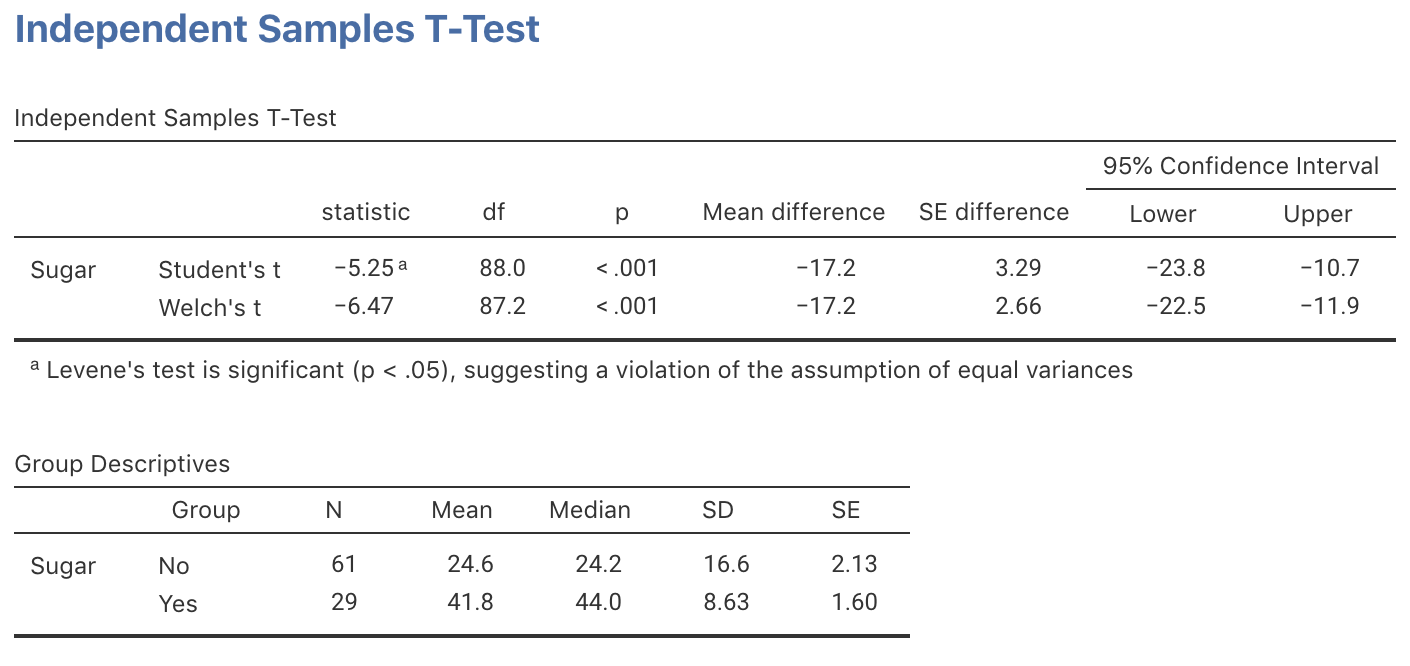## 30.12 Exercises

Selected answers are available in Sect. D.28.

Exercise 30.1 Earlier, the NHANES study (Sect. 12.10; Exercise 24.1), was used to address this RQ:

Among Americans, is the mean direct HDL cholesterol different for current smokers and non-smokers?

Use the SPSS output in Fig. 30.5 to perform a hypothesis test to answer the RQ.FIGURE 30.5: SPSS output for the NHANES data

Exercise 30.2 A study of male paramedics in Western Australia compared conventional paramedics with special operations paramedics . Some information comparing their physical profiles is shown in Table 30.5.

1. Compute the missing standard errors.
2. Consider comparing the mean grip strength for the two groups of paramedics. The standard error for the difference between the means is 3.3044.
• Carefully write down the hypotheses.
• Compute the $$t$$-score for testing if a difference exists between the two types of paramedics.
• Approximate the $$P$$-value using the 68–95–99.7 rule.
• Discuss the conditions required for statistical validity in this context.
• Make a conclusion.
3. Consider comparing the mean number of push-ups completed in one minute. The standard error for the difference between the means is 4.0689.
• Carefully write down the hypotheses.
• Compute the $$t$$-score for testing if a difference exists between the two types of paramedics.
• Approximate the $$P$$-value using the 68–95–99.7 rule.
• Discuss the conditions required for statistical validity in this context.
• Make a conclusion.
TABLE 30.5: The physical profile of conventional ($$n=18$$) and special operation ($$n=11$$) paramedics in Western Australia
Conventional Special Operations
Grip strength (in kg)
Mean 51 56
Std deviation 8 9
Std error
Push-ups (per minutes)
Mean 36 47
Std deviation 10 11
Std error

Exercise 30.3 Consider again the body temperature data from Sect. 27.1. The researchers also recorded the gender of the patients, as they also wanted to compare the mean internal body temperatures for males and females.

Use the jamovi output in Fig. 30.6 to perform this test and make a conclusion. Also comment on the practical significance of your results.FIGURE 30.6: jamovi output for the body-temperature data

Exercise 30.4 A study examined the sugar consumption in industrialised (mean: 41.8 kg/person/year) and non-industrialised (mean: 24.6 kg/person/year) countries. The jamovi output is shown in Fig. 30.7.

1. Write the hypotheses.
2. Write down and interpret the CI.
3. Write a conclusion for the hypothesis test.FIGURE 30.7: jamovi output for the sugar-consumption data

In the image below, click on the location where the correct $$P$$-value is located.

### References

Chapman D, Peiffer J, Abbiss CR, Laursen PB. A descriptive physical profile of Western Australian male paramedics. Journal of Emergency Primary Health Care. 2007;5(1).
Woodward M, Walker ARP. Sugar consumption and dental caries: Evidence from 90 countries. British Dental Journal. 1994;176:297–302.